# Picture 2

Picture free is of length 14.2 cm and breadth 10.4 cm. The frame has border of uniforms with 2.6 cm. Find the area of photograph.

A =  46.8 cm2

### Step-by-step explanation: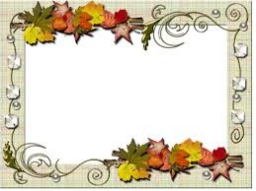Did you find an error or inaccuracy? Feel free to write us. Thank you!#### You need to know the following knowledge to solve this word math problem:

We encourage you to watch this tutorial video on this math problem:

## Related math problems and questions:

• Frame image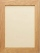Peter wants to put a picture in a frame. The picture is 7 3/5 cm wide. To fit in the picture frame cannot be more than 7 3/10 cm wide. How much should the image be trimmed?
• PrismCalculate the surface area and volume of a prism with a body height h = 10 cm, and its base has the shape of a rhomboid with sides a = 5.8 cm, b = 3 cm, and the distance of its two longer sides is w = 2.4 cm.
• Area and perimeter 2Find the area and the perimeter of a rectangle of length 45 1/2 cm and breadth 16 2/3 cm.
• Photo frameA square shape photo with a side length of 20 cm is framed by a 4 cm wide bar. Find the external frame size of this photo (the frame is from all sides)
• Similar trianglesThe triangles ABC and XYZ are similar. Find the missing lengths of the sides of the triangles. a) a = 5 cm b = 8 cm x = 7.5 cm z = 9 cm b) a = 9 cm c = 12 cm y = 10 cm z = 8 cm c) b = 4 cm c = 8 cm x = 4.5 cm z = 6 cm
• Colored paperCan a 4 cm, 3 cm and 2 cm block be glued with a colored quarter of paper measuring 7 cm and 8 cm?
• Rectangles - sidesOne side of the rectangle is 10 cm longer than second. Shortens longer side by 6 cm and extend shorter by 14 cm increases the area of the rectangle by 130 cm2. What are the dimensions of the original rectangle?
• Prism 4 sidesFind the surface area and volume four-sided prism high 10cm if its base is a rectangle measuring 8 cm and 1.2dm
• The carpet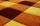How many meters of carpet 90 cm wide need to cover floor room which has a rectangular shape with a lengths 4.8 m and 2.4 m if the number of pieces on the carpet is needed to be lowest?
• 2d shapeCalculate the content of a shape in which an arbitrary point is not more than 3 cm from the segment AB. The length of the segment AB is 5 cm.
• Largest wallFind the content of the largest wall of a prism with a rectangle base with a height of 4 dm, side c = 5 cm, and side b = 6 cm.
• Parallerogram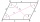In parallelogram ABCD is a = 2.5 cm and height to side a is 7.2 cm and height to side b is 4 cm. Find the side length b.
• RectangleThe length of the rectangle is 12 cm greater than 3 times its width. What dimensions and area this rectangle has if ts circumference is 104 cm.
• A photographA photograph will stick to a white square letter with a x cm length. The photo is 3/4 x cm long and 20 cm wide than the width of the paper. The surface of the remaining paper surrounding the photograph is 990 cm2. Find the size of paper and photo.
• Do you solve this?Determine area S of rectangle and length of its sides if its perimeter is 102 cm.
• Minimum surfaceFind the length, breadth, and height of the cuboid-shaped box with a minimum surface area, into which 50 cuboid-shaped blocks, each with length, breadth, and height equal to 4 cm, 3 cm, and 2 cm respectively, can be packed.
• Hřiště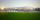Map scale is 1: 5000. The playground is rectangular and on the map has dimensions 10 cm and 5 cm What is area of playground in square meters in reality?